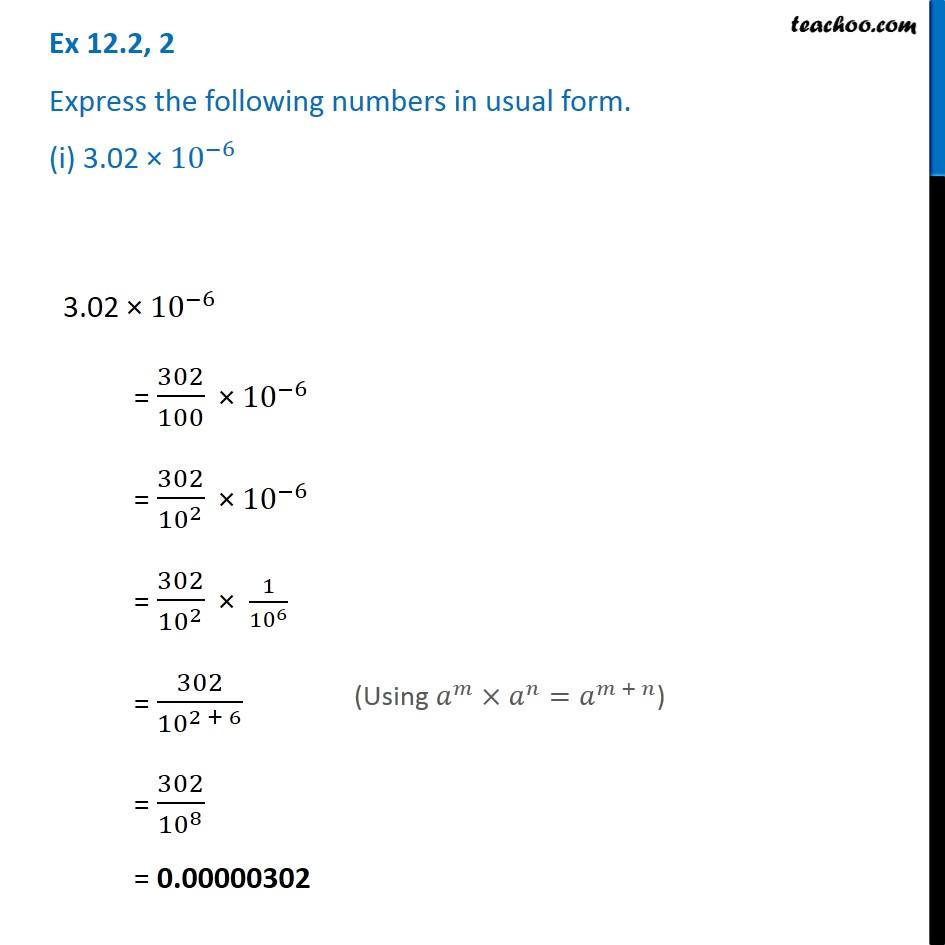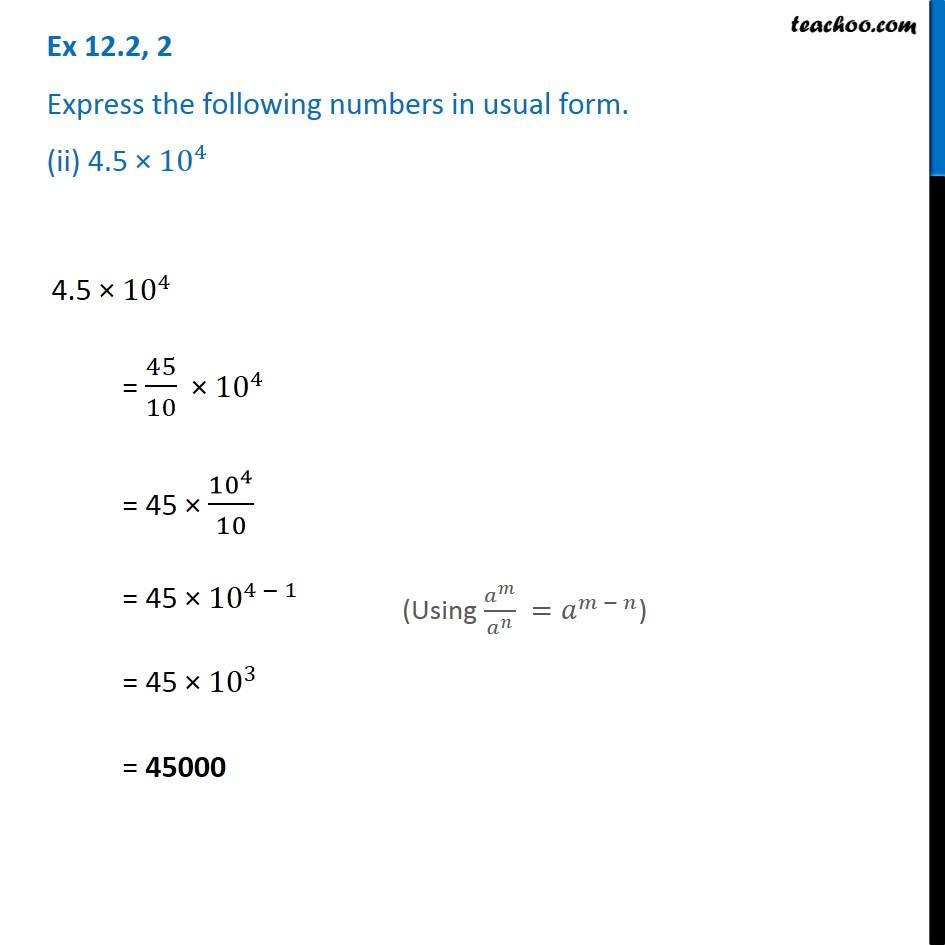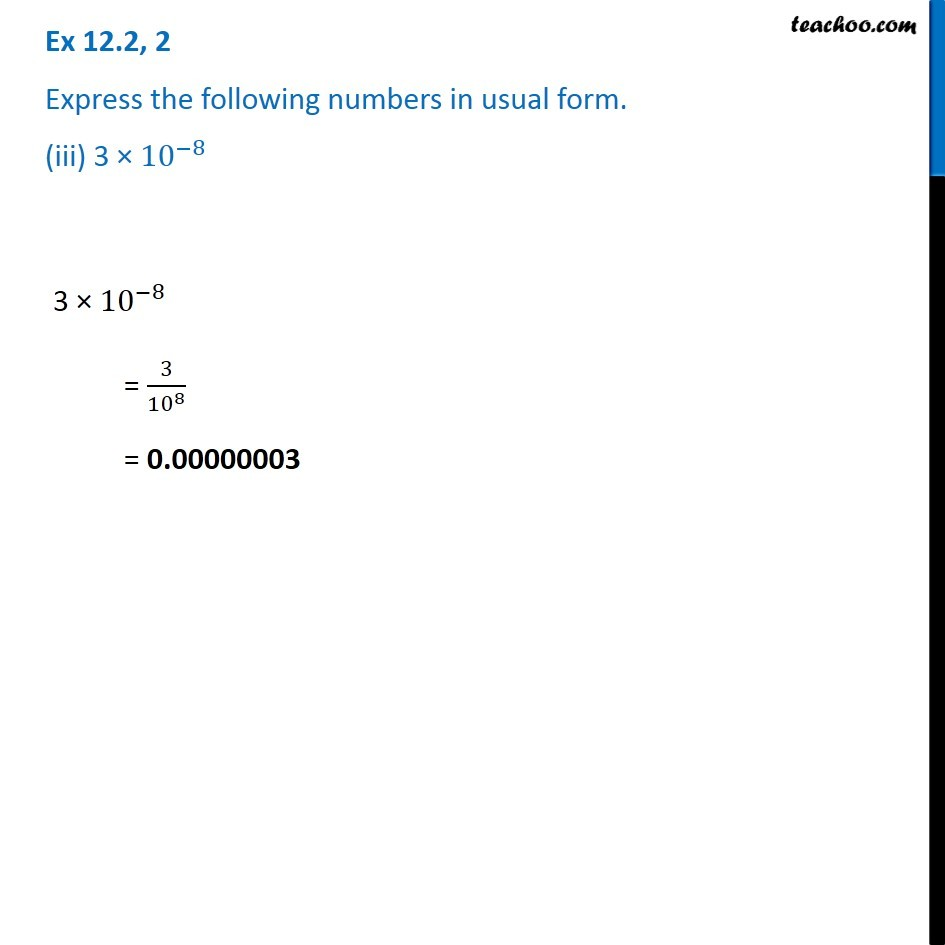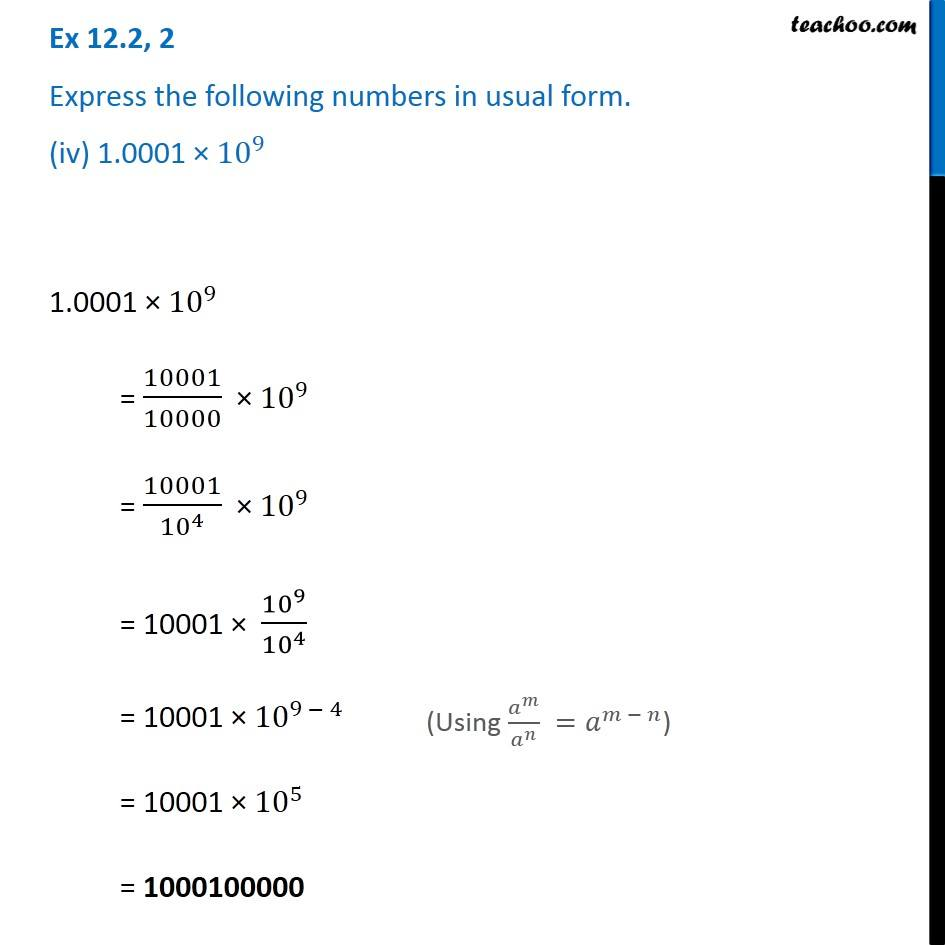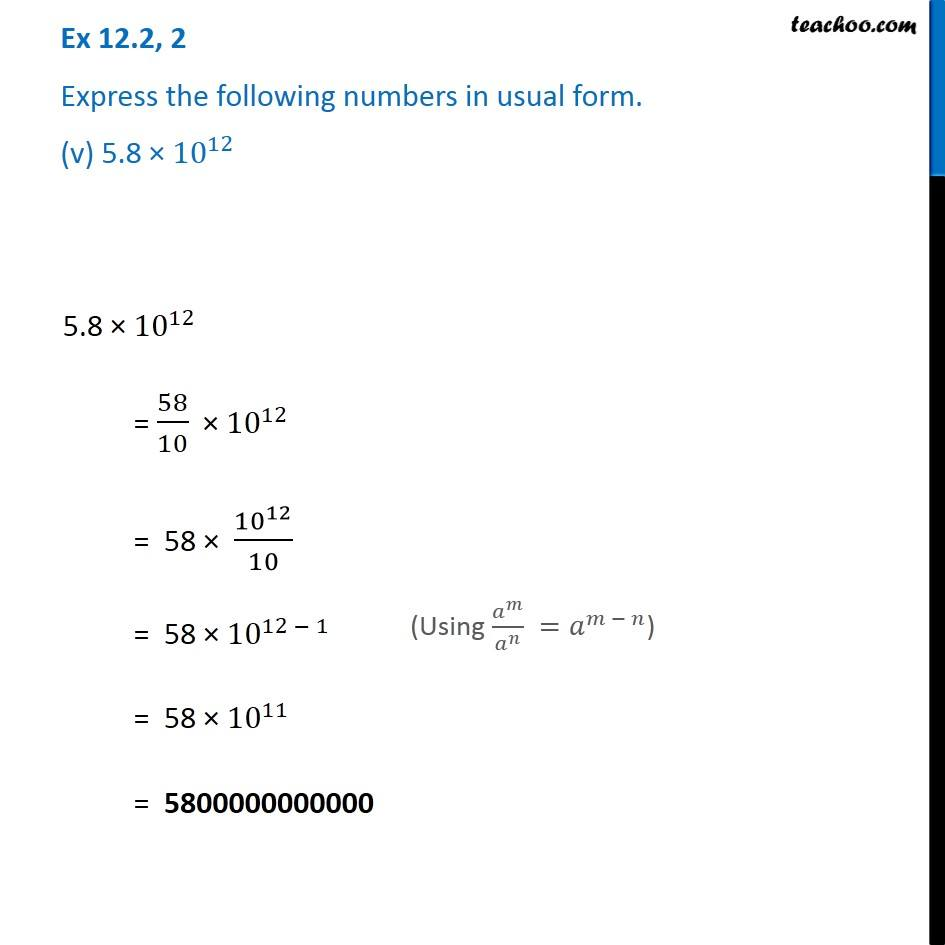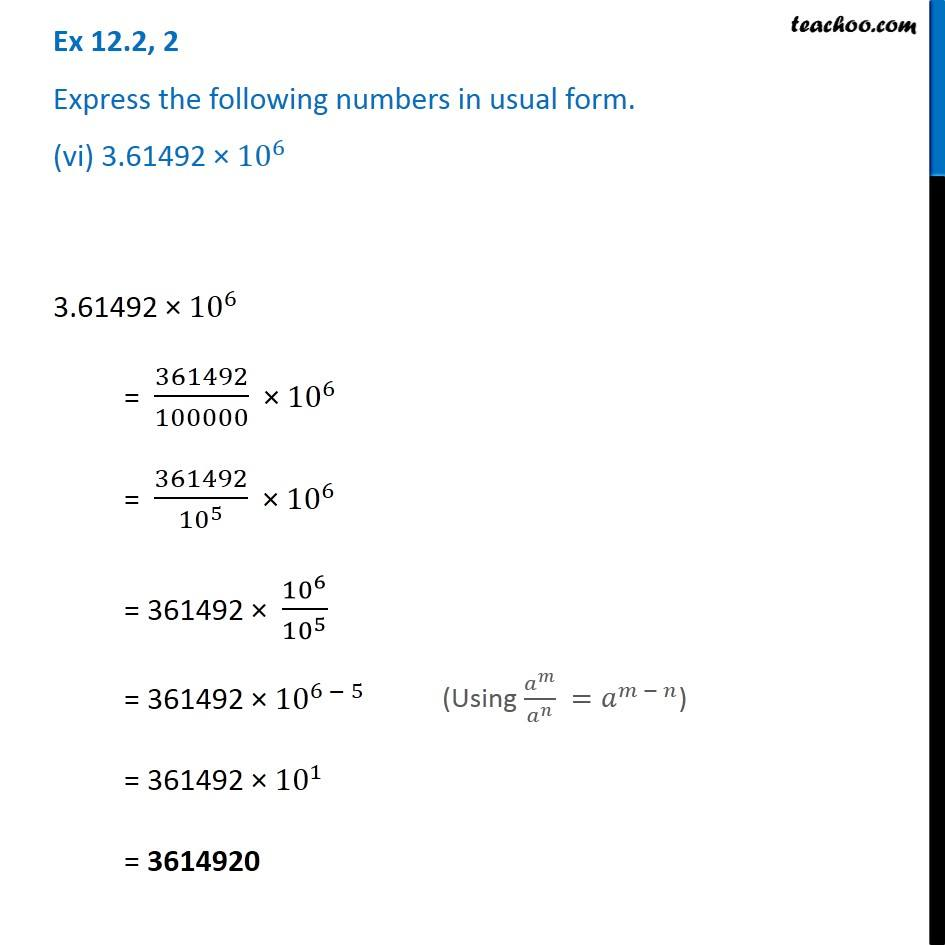Subscribe to our Youtube Channel - https://you.tube/teachoo

1. Chapter 12 Class 8 Exponents and Powers
2. Serial order wise
3. Ex 12.2

Transcript

Ex 12.2, 2 Express the following numbers in usual form. (i) 3.02 × 〖10〗^(−6) 3.02 × 〖10〗^(−6) = 302/100 × 〖10〗^(−6) = 302/〖10〗^2 × 〖10〗^(−6) = 302/〖10〗^2 × 1/〖10〗^6 = 302/〖10〗^(2 + 6) = 302/〖10〗^8 = 0.00000302 (Using 𝑎^𝑚×𝑎^𝑛=𝑎^(𝑚 + 𝑛)) Ex 12.2, 2 Express the following numbers in usual form. (ii) 4.5 × 〖10〗^4 4.5 × 〖10〗^4 = 45/10 × 〖10〗^4 = 45 × 〖10〗^4/10 = 45 × 〖10〗^(4 − 1) = 45 × 〖10〗^3 = 45000 (Using 𝑎^𝑚/𝑎^𝑛 =𝑎^(𝑚 − 𝑛)) Ex 12.2, 2 Express the following numbers in usual form. (iii) 3 × 〖10〗^(−8) 3 × 〖10〗^(−8) = 3/〖10〗^8 = 0.00000003 Ex 12.2, 2 Express the following numbers in usual form. (iv) 1.0001 × 〖10〗^9 1.0001 × 〖10〗^9 = 10001/10000 × 〖10〗^9 = 10001/〖10〗^4 × 〖10〗^9 = 10001 × 〖10〗^9/〖10〗^4 = 10001 × 〖10〗^(9 − 4) = 10001 × 〖10〗^5 = 1000100000 (Using 𝑎^𝑚/𝑎^𝑛 =𝑎^(𝑚 − 𝑛)) Ex 12.2, 2 Express the following numbers in usual form. (v) 5.8 × 〖10〗^12 5.8 × 〖10〗^12 = 58/10 × 〖10〗^12 = 58 × 〖10〗^12/10 = 58 × 〖10〗^(12 − 1) = 58 × 〖10〗^11 = 5800000000000 (Using 𝑎^𝑚/𝑎^𝑛 =𝑎^(𝑚 − 𝑛)) Ex 12.2, 2 Express the following numbers in usual form. (vi) 3.61492 × 〖10〗^6 3.61492 × 〖10〗^6 = 361492/100000 × 〖10〗^6 = 361492/〖10〗^5 × 〖10〗^6 = 361492 × 〖10〗^6/〖10〗^5 = 361492 × 〖10〗^(6 − 5) = 361492 × 〖10〗^1 = 3614920 (Using 𝑎^𝑚/𝑎^𝑛 =𝑎^(𝑚 − 𝑛))

Ex 12.2

About the AuthorDavneet Singh
Davneet Singh is a graduate from Indian Institute of Technology, Kanpur. He has been teaching from the past 9 years. He provides courses for Maths and Science at Teachoo.